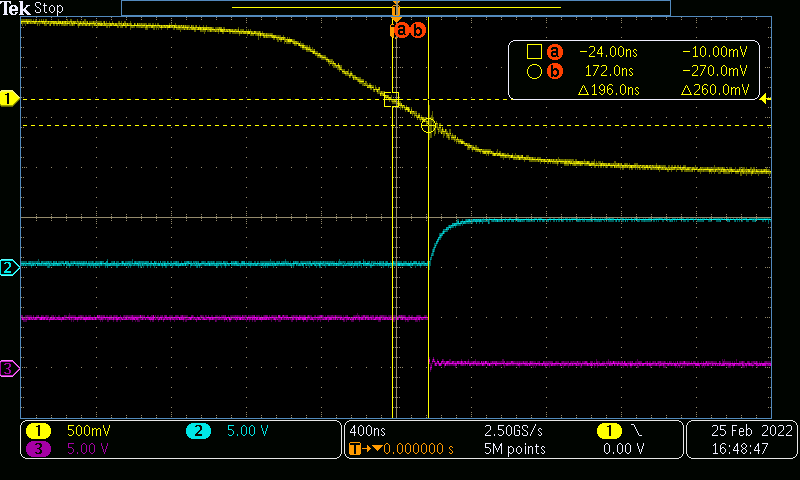SBAA542 March   2022

Design Goals

High

Side Supply

Input

Voltage

Working Voltage

Low Side Supply

Output Voltage

12 V

±170 Vpk Sine Wave

≥ 400 VRMS

3.3 V to 5.0 V ±10%

≤ Low-Side Supply

Design Description

A zero-crossing detector circuit changes output state when the AC input crosses the zero-cross reference voltage. This design features a single chip solution for zero-crossing detection of an AC sine wave with inverting and non-inverting digital outputs. The circuit is created by setting the comparator inverting input to ground and applying a clamped sine wave to the noninverting input. The input voltage is clamped by R1 and a pair of antiparallel diodes. In this case, diodes are used instead of an attenuator to maximize the slew rate of the input near the zero-crossing, which reduces output latency. The circuit is used for AC line zero-cross detection in control circuits to reduce standby and off-mode power consumption.

Isolated Zero-Cross Detection Circuit Schematic

Design Notes

1. The circuit must be capable of handling 750-V working voltage across the isolation barrier.
2. The maximum input voltage at IN+ must be ±1 V
3. Inverting and non-inverting output are desired
4. Maximum current flowing through R1 is 100 µA ±10%
5. Limit the operating voltage of each resistor in the string to 100 V ±10% maximum
6. The input AC source voltage is 120 VRMS, higher AC voltages are easily accommodated with component modifications. See the Alternate Design section for details
7. Ensure the hysteresis voltage at the AC zero-cross is no more than ±30 mV

Design Steps

1. Determine the ideal R1 resistor value. The maximum peak input voltage of 120 VRMS × √2 = 170 VPK. Note that the forward voltage of the diode D1 is near zero, and not included in this calculation.
2. Divide R1 into 3 equal resistors to maintain design limits of ≤ 100 V per resistor:
3. Use the Analog Engineer’s Calculator to find a standard E96 1% resistor value for R1. The nearest value is 569 kΩ.
4. Select the anti-parallel diodes. Choose diodes which will provide at least ± 350-mV forward voltage with the 100 µA supplied through R1.
5. Optional – design low-pass filter at VINP defined by R2 and C1. The frequency response is defined as:
${F}_{C}=\frac{1}{2\pi ×R2×C1}$

Revised Design

The following schematic shows implementation of the revised design using the AMC23C10.

Revised Design With AMC23C10 Isolated Comparator

The AMC23C10 uses capacitive isolation to provide a working voltage of 1000 V. The voltage source for VDD1 is specified from 3 V to 27 V, controlled internally through an LDO. VDD2 is specified from 2.7 V to 5.5 V. The input voltage range under normal operation is ±1 V. The logic output on OUT1 is open drain which can be used with a pullup resistor to VDD1. OUT2 is a push-pull type output needing no external pullup resistors.

Design Simulations

Simulation of Zero-Crossing Detection With Sine Wave Input
Simulation of Zero-Crossing Detection With Rectified Input
Simulation of Response Time for Zero-Crossing Detection

Measured Response

The following images show the measured response of the zero-crossing detection circuit using the AMC23C10 isolated comparator. The input is captured on trace 1, while OUT1 and OUT2 are shown on traces 2 and 3 respectively. When measured at both the rising and falling edges of the input, the delay between the zero-crossing of the input and the output transition does not exceed 220 ns.Zero-Crossing Detection of Rectified InputZero-Crossing Detection Output Latency – Falling EdgeZero-Crossing Detection Output Latency – Rising Edge

Design References

See Analog Engineer's Circuit Cookbooks for TI's comprehensive circuit library.

Texas Instruments, AMC23C10 Fast Response, Reinforced Isolated Comparator With Dual Output data sheet

Design Featured Isolated Comparator

AMC23C10
Working Voltage 1000 VRMS
VDD1 3.0 V–27 V
VDD2 2.7 V–5.5 V
Input Voltage Range ±1000 mV
Output Options OUT1 - Open Drain
OUT2 - Push-Pull
AMC23C10

Alternate Design for 230-VAC Input

AMC23C10
Working Voltage 1000 VRMS
AC Input 325 Vpk
R1 Ideal 3.25 MΩ
R1 E96 Standard Three each 1.09 MΩ